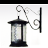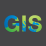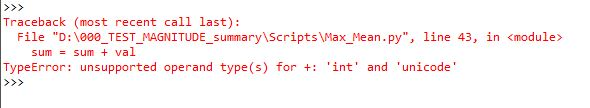# Getting the Mean value out of the Max values of a raster data set

1527
15
11-27-2018 03:41 AMOccasional Contributor II

Hi,

As the title says, I'm trying to calculate in python the mean value out of all maximum values in a list of rasters (696) in a GDB.

First thing would be to calculate all max values but I'm not sure how to "hold" all those values to then calculate the mean.

Any help will be greatly appreciate it.

Running ArcGis 10.5.1

Tags (3)
1 Solution

Accepted SolutionsbyEsri Esteemed Contributor

Change int to float:

``````# Iterate through each raster and append max val to list
for raster in arcpy.ListRasters("*"):
val = arcpy.GetRasterProperties_management(raster, "MAXIMUM")
maxVal = float(val.getOutput(0))
maxVals.append(maxVal)‍‍‍‍‍``````
15 RepliesbyEsri Esteemed Contributor

I'm not entirely sure what you want to achieve.  When you mention you want to calculate the maximum, do you refer to a single value for the raster (like the metadata property of the raster) or the maximum for each pixel over the series of rasters or the maximum in a certain neighborhood (moving window) for each raster. And the mean would be using the values of each pixel for the series of maximum rasters?

You can create temp rasters using the in_memory workspace if they are not to big in case you do not want to create a physical result for the intermediate max rasters.New Contributor III

Basically you would iterate over the rasters, find the maximum, add the result to array, and at the end you would calculate mean of the values in array.

You could use Get Raster Properties function to get the maximum of the raster.Occasional Contributor II

Thanks Xander and Martin for your suggestions.

Sorry Xander I may have not explained the issue correctly.

My ultimate goal here is to standardise a number of raster layers (696 of them). My first objective was to get the maximum value (a unique single value) for all those rasters. I've done that; but after examining the maximum value I realise that doing the mean of all the maximum values of all rasters would be a more accurate value (to later standardise them).

What Martin says is what I need to do but I'm not familiar with numpy and arrays and I'm not quite sure how to proceed. I also wonder if there is a function in scipy that would do that directly? Below is just the start of the script but as I said I don't know how to proceed.

``````import arcpy
import math
import os
import numpy
import scipy
from arcpy import env
arcpy.env.overwriteOutput=True
from arcpy.sa import*
arcpy.CheckOutExtension("Spatial")

env.workspace = r"D:\000_TEST_MAGNITUDE_summary\MAX_VAL_calculation\Vel_rasters.gdb

# List rasters and get MAX values

rasterlist1 = arcpy.ListRasters()

Vel_max = arcpy.GetRasterProperties_management(rasterlist1, "MAXIMUM")

# Create array and add result from above

Vel_array = arcpy.RasterToNumPyArray(rasterlist1)‍‍‍‍‍‍‍‍‍‍‍‍‍‍‍‍‍‍‍‍‍‍‍‍‍‍``````byMVP Esteemed Contributor

scipy relies on numpy, see my second example, it returns a list of the maximums, then simply takes the mean of thatbyEsri Esteemed Contributor

You could try the following:

``````import arcpy
arcpy.env.workspace = r"C:\TEMP\PYTHON\Data.gdb"

# Create list
maxVals = []

# Iterate through each raster and append max val to list
for raster in arcpy.ListRasters("*"):
val = arcpy.GetRasterProperties_management(raster, "MAXIMUM")
maxVal = int(val.getOutput(0))
maxVals.append(maxVal)

# Sum list
sum = 0
for val in maxVals:
sum += val

# Find Mean
meanVal = sum / len(maxVals)
print(meanVal)‍‍‍‍‍‍‍‍‍‍‍‍‍‍‍‍‍‍‍‍``````Occasional Contributor II

Thank you Jake. That looks promising. Just trying now but I got an error in line 16. Is it '+' or '='?byEsri Esteemed Contributor

It's actually +=.  It's essentially a quicker way of writing:

``sum = sum + val‍``Occasional Contributor II

I see. At first run I got an error in line 13 below. As the rasters are floating point I removed 'int' from the line (as below).

``````env.workspace = r"D:\000_TEST_MAGNITUDE_summary\MAX_VAL_calculation\Vel_rasters.gdb"

# Create list

maxVals = []

# Iterate through each raster and append max val to list

for raster in arcpy.ListRasters("*"):
val = arcpy.GetRasterProperties_management(raster, "MAXIMUM")
maxVal = val.getOutput(0)
maxVals.append(maxVal)

# Sum list

sum = 0
for val in maxVals:
sum = sum + val

# Find Mean

meanVal = sum / len(maxVals)

print(meanVal)‍‍‍‍‍‍‍‍‍‍‍‍‍‍‍‍‍‍‍‍‍‍‍‍‍‍‍‍‍‍‍‍‍‍‍‍‍‍‍‍‍‍‍‍‍‍‍‍‍‍‍‍‍‍‍``````

But now I'm getting this error which I guess it may be related.byEsri Esteemed Contributor

Change int to float:

``````# Iterate through each raster and append max val to list
for raster in arcpy.ListRasters("*"):
val = arcpy.GetRasterProperties_management(raster, "MAXIMUM")
maxVal = float(val.getOutput(0))
maxVals.append(maxVal)‍‍‍‍‍``````